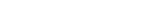MathExamle.com# Multiplication and Division of integers

Create worksheets of mathematical examples with multiplication and division of integers, select type of a generator and viewing some samples of task

Creates examples with multiplication and division integers

6 Variants 4 Levels
 8÷2= 6×(8÷2)÷3= 8÷2= 16÷4×7= 12÷(9÷3)×7= 12×3=
Variant: A Level: Easy

Creates examples with a multiplication operation of integers by a column method

1 Variant 5 Levels
 × 81 4
 × 31 7
 × 48 4
 × 27 4
Variant: 1 Level: Light

Creates examples with a division operation of integers by a column method

1 Variant 5 Levels
 96000÷40= 18732÷28= 29750÷17= 22400÷32= 71766÷81= 37200÷10=
Variant: 1 Level: Difficult

Creates examples with a long division of integers according to the British system

1 Variant 5 Levels
 12 9000
 71 8449
 31 8897
Variant: 1 Level: Normal

Creates examples with a division operation, that can contain the remainder of the division

1 Variant 3 Levels
 45÷18= 151÷12= 90÷6= 26÷4= 111÷11= 108÷18=
Variant: 1 Level: Normal

Creates examples with a long division according to the British system, that can contain the remainder of the division

1 Variant 3 Levels
 3 29
 5 41
 7 41
 7 20
Variant: 1 Level: Easy